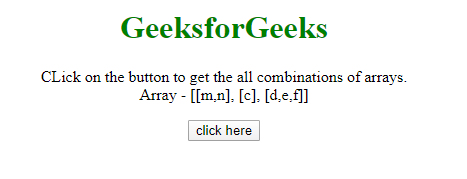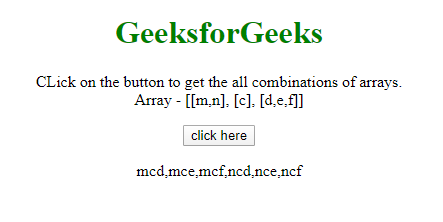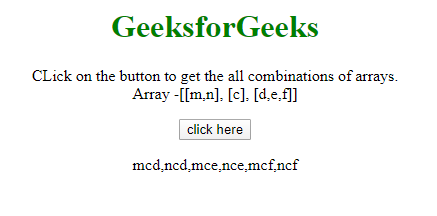# Find all the combinations of the array values in JavaScript

The task is to get the cartesian product of the arrays(Finding the all combination after concatenating them). Here, 2 approaches are discussed with the help of JavaScript.

Approach 1:

• Get the all arrays in an array.
• Recursion is used to solve the problem. The base condition is, When the length of the array reduces to zero then return the string build till now. Else
• Reduce the first array element by .reduce() method and return the result returned from the recursion result(Recursion is called every time after leaving the first item of the array) plus the previous value in concatenation with every array element.
• Return the final ans array, which contains the all combinations.

Example 1: This example implements the above approach.

 ` ` `<``html``> ` ` `  `<``head``> ` `    ``<``title``> ` `        ``All combinations of a JavaScript array values. ` `    `` ` ` ` ` `  `<``body` `style``=``"text-align:center;"``> ` `    ``<``h1` `style``=``"color:green;"``>    ` `            ``GeeksforGeeks    ` `        `` ` `    ``<``p` `id``=``"GFG_UP"``> ` `    `` ` `    ``<``button` `id``=``"button"` `onclick``=``"GFG_Fun();"``> ` `        ``click here ` `    `` ` `    ``<``p` `id``=``"GFG_DOWN"``> ` `    `` ` `    ``<``script``> ` `        ``var up = document.getElementById('GFG_UP'); ` `        ``var down = document.getElementById('GFG_DOWN'); ` `        ``var arr = [ ` `            ``['m', 'n'], ` `            ``['c'], ` `            ``['d', 'e', 'f'] ` `        ``]; ` `        ``up.innerHTML = "CLick on the button to get the "+ ` `          ``"all combinations of arrays.<``br``>Array - "+ ` `          ``"[[" + arr + "], [" + arr + "], [" + arr + "]]"; ` ` `  `        ``function getCombn(arr, pre) { ` `            ``pre = pre || ''; ` `            ``if (!arr.length) { ` `                ``return pre; ` `            ``} ` `            ``var ans = arr.reduce(function(ans, value) { ` `                ``return ans.concat(getCombn(arr.slice(1), pre + value)); ` `            ``}, []); ` `            ``return ans; ` `        ``} ` ` `  `        ``function GFG_Fun() { ` `            ``down.innerHTML = getCombn(arr); ` `        ``} ` `    `` ` ` ` ` `  ` `

Output:

• Before clicking on the button:• After clicking on the button:Approach 2:

• Get the all arrays in an array.
• Recursion is used to solve the problem. The base condition is, When the length of the array reduces to one then return that element of the array. Else
• Call recursion after leaving the first element of the array and store the result in variable(otherCases).
• Loop through every element of the Array(otherCases) and inside every element loop through the first element of the Array(arr).
• Concatenate every element of the Array(arr) with the Array(otherCases) and push the result in answer array.

Example 2: This example implements the above approach.

 ` ` `<``html``> ` ` `  `<``head``> ` `    ``<``title``> ` `        ``all combinations of a JavaScript array values. ` `    `` ` ` ` ` `  `<``body` `style``=``"text-align:center;"``> ` `    ``<``h1` `style``=``"color:green;"``>    ` `            ``GeeksforGeeks    ` `        `` ` `    ``<``p` `id``=``"GFG_UP"``> ` `    `` ` `    ``<``button` `id``=``"button"` `onclick``=``"GFG_Fun();"``> ` `        ``click here ` `    `` ` `    ``<``p` `id``=``"GFG_DOWN"``> ` `    `` ` `    ``<``script``> ` `        ``var up = document.getElementById('GFG_UP'); ` `        ``var down = document.getElementById('GFG_DOWN'); ` `        ``var arr = [ ` `            ``['m', 'n'], ` `            ``['c'], ` `            ``['d', 'e', 'f'] ` `        ``]; ` `        ``up.innerHTML = "CLick on the button to get the all "+ ` `          ``"combinations of arrays.<``br``>Array -"+ ` `          ``"[[" + arr + "], [" + arr + "], [" + arr + "]]"; ` ` `  `        ``function getCombn(arr) { ` `            ``if (arr.length == 1) { ` `                ``return arr; ` `            ``} else { ` `                ``var ans = []; ` `               `  `                ``// recur with the rest of the array. ` `                ``var otherCases = getCombn(arr.slice(1));  ` `                ``for (var i = 0; i < ``otherCases.length``; i++) { ` `                    ``for (var ``j` `= ``0``; j < arr.length; j++) { ` `                        ``ans.push(arr[j] + otherCases[i]); ` `                    ``} ` `                ``} ` `                ``return ans; ` `            ``} ` `        ``} ` ` `  `        ``function GFG_Fun() { ` `            ``down.innerHTML` `= ``getCombn``(arr); ` `        ``} ` `    `` ` ` ` ` `  ` `

Output:

• Before clicking on the button:• After clicking on the button:My Personal Notes arrow_drop_upCheck out this Author's contributed articles.

If you like GeeksforGeeks and would like to contribute, you can also write an article using contribute.geeksforgeeks.org or mail your article to contribute@geeksforgeeks.org. See your article appearing on the GeeksforGeeks main page and help other Geeks.

Please Improve this article if you find anything incorrect by clicking on the "Improve Article" button below.

Article Tags :

Be the First to upvote.

Please write to us at contribute@geeksforgeeks.org to report any issue with the above content.